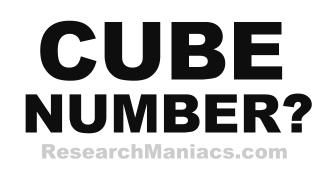Is 19 a cube number?

Cube Question: Is 19 a cube number?

For 19 to be a cube number the following equation must be true:

n3 = 19 where n is a whole number.

In other words, if n*n*n = 19, then 19 is a cube number.

There are no whole numbers you can set to n to make the equation above 19. Therefore, we can conclude that 19 is NOT a cube number.

Answer: Is 19 a cube number? = NO

 Cube Number? Check other numbers to see if they are cube. List of Cube Numbers Here is a list of cube numbers.